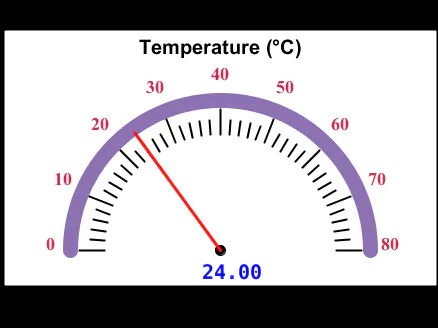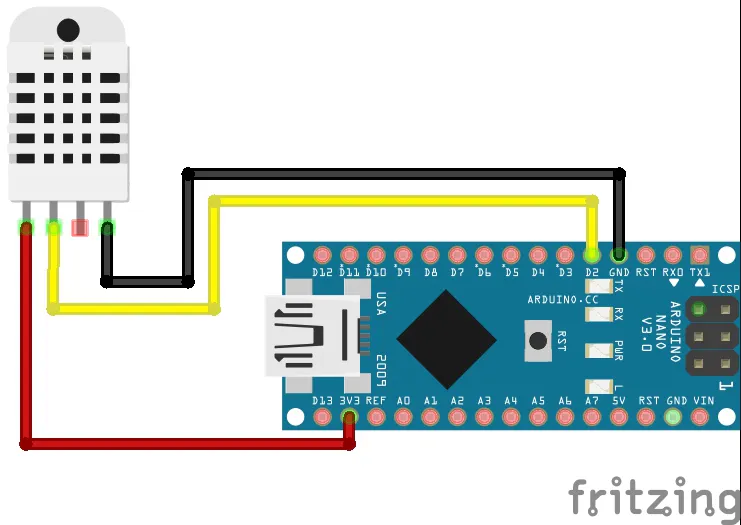# Create GUI To Visualize Temp And Humidity For Arduino

Simplest way to create a Graphical User Interface (GUI) to visualize Temperature and Humidity using Arduino and DHT sensor in Processing.

BeginnerFull instructions provided30 minutes23,159## Things used in this project

### Hardware components

 Arduino Nano
×1
 DHT22
×1×1
 Jumper Wires
×1

### Software apps and online servicesArduino IDE
 Processing

## Schematics

### Schematic

You can use 5 V also## Code

### Arduino code

Arduino
You need 'SimpleDHT' library to run this code successfully. Just go to library manager and search for 'SimpleDHT'. Very simple, Right?
```// Download 'SimpleDHT' library from the library manager to run this
// code successfully.
#include <SimpleDHT.h>

// for DHT22,
//      VCC: 5V or 3V
//      GND: GND
//      DATA: 2
int pinDHT22 = 2;
SimpleDHT22 dht22;

void setup() {
Serial.begin(9600);
}

void loop() {
// start working...

// @remark We use read2 to get a float data, such as 10.1*C
//    if user doesn't care about the accurate data, use read to get a byte data, such as 10*C.
float temperature = 0;
float humidity = 0;
int err = SimpleDHTErrSuccess;
if ((err = dht22.read2(pinDHT22, &temperature, &humidity, NULL)) != SimpleDHTErrSuccess) {
return;
}

Serial.print((float)temperature);
Serial.print(",");
Serial.println((float)humidity);

// DHT22 sampling rate is 0.5HZ.
delay(2500);
}
```

### Processing code

Processing
```/***********************************
First upload the Arduino code to the Arduino and then run this code.
Make sure that the Port number and baudrate are the same.
***********************************/
import meter.*;
import processing.serial.*;

Serial port;
String[] list;

Meter m, m2;

void setup() {
size(950, 400);
background(0);

port = new Serial(this, "COM4", 9600);

fill(120, 50, 0);
m = new Meter(this, 25, 100);
// Adjust font color of meter value
m.setTitleFontSize(20);
m.setTitleFontName("Arial bold");
m.setTitle("Temperature (C)");
m.setDisplayDigitalMeterValue(true);

// Meter Scale
String[] scaleLabelsT = {"0", "10", "20", "30", "40", "50", "60", "70", "80"};
m.setScaleLabels(scaleLabelsT);
m.setScaleFontSize(18);
m.setScaleFontName("Times New Roman bold");
m.setScaleFontColor(color(200, 30, 70));

m.setArcColor(color(141, 113, 178));
m.setArcThickness(10);
m.setMaxScaleValue(80);

m.setNeedleThickness(3);

m.setMinInputSignal(0);
m.setMaxInputSignal(80);

// A second meter for reference
int mx = m.getMeterX();
int my = m.getMeterY();
int mw = m.getMeterWidth();

m2 = new Meter(this, mx + mw + 20, my);
m2.setTitleFontSize(20);
m2.setTitleFontName("Arial bold");
m2.setTitle("Humidity (%)");
m2.setDisplayDigitalMeterValue(true);

String[] scaleLabelsH = {"0", "10", "20", "30", "40", "50", "60", "70", "80", "90", "100"};
m2.setScaleLabels(scaleLabelsH);
m2.setScaleFontSize(18);
m2.setScaleFontName("Times New Roman bold");
m2.setScaleFontColor(color(200, 30, 70));

m2.setArcColor(color(141, 113, 178));
m2.setArcThickness(10);
m2.setMaxScaleValue(100);

m2.setNeedleThickness(3);

m2.setMinInputSignal(0);
m2.setMaxInputSignal(100);

}

public void draw() {

textSize(30);
fill(0, 255, 0);
text("Temperature and Humidity", 270, 50);

if (port.available() > 0) {
list = split(val, ',');
float temp = float(list);
float hum = float(list);

println("Temperature: " + temp + " C  " + "Humidity: " + hum + " %");

m.updateMeter(int(temp));
m2.updateMeter(int(hum));
}
}

/*
// Arduino code

#include <SimpleDHT.h>

// for DHT22,
//      VCC: 5V or 3V
//      GND: GND
//      DATA: 2
int pinDHT22 = 2;
SimpleDHT22 dht22;

void setup() {
Serial.begin(9600);
}

void loop() {
// start working...

// @remark We use read2 to get a float data, such as 10.1*C
//    if user doesn't care about the accurate data, use read to get a byte data, such as 10*C.
float temperature = 0;
float humidity = 0;
int err = SimpleDHTErrSuccess;
if ((err = dht22.read2(pinDHT22, &temperature, &humidity, NULL)) != SimpleDHTErrSuccess) {
return;
}

Serial.print((float)temperature);
Serial.print(",");
Serial.println((float)humidity);

// DHT22 sampling rate is 0.5HZ.
delay(2500);
}
*/
```

### Processing code

Processing
```// Lets create a simple meter

// Import Meter library
import meter.*;

// Import serial library
import processing.serial.*;

Serial port; // Define a port

Meter m, m2;

void setup(){
// First we need to create a empty window
size(950, 400); // Size of the window (width, height)
background(0, 0, 0); // Background color of window (R,G,B)

// Create new port
port = new Serial(this, "COM3", 9600); //name of the port would be different for linux

// Lets add a default meter to empty window
// TEMPERATURE METER
m = new Meter(this, 25, 80);  // here 25, 10 are x and y coordinates of meter's upper left corner

m.setTitleFontSize(20);
m.setTitleFontName("Arial bold");
m.setTitle("Temperature (C)");

// Change meter scale values
String[] scaleLabels = {"0", "10", "20", "30", "40", "50", "60", "70", "80"};
m.setScaleLabels(scaleLabels);
m.setScaleFontSize(18);
m.setScaleFontName("Times new roman bold");
m.setScaleFontColor(color(200, 30, 70));

// We can also display the value of meter
m.setDisplayDigitalMeterValue(true);

// Lets do some more modifications so our meter looks nice
m.setArcColor(color(141, 113, 178));
m.setArcThickness(15);

m.setMaxScaleValue(80);

m.setMinInputSignal(0);
m.setMaxInputSignal(80);

m.setNeedleThickness(3);

// HUMIDITY METER
// lets take some refference from first meter
int mx = m.getMeterX(); // x coordinate of m
int my = m.getMeterY(); // y coordinate of m
int mw = m.getMeterWidth();

m2 = new Meter(this, mx + mw + 20, my);

m2.setTitleFontSize(20);
m2.setTitleFontName("Arial bold");
m2.setTitle("Humidity (%)");

// Change meter scale values
String[] scaleLabels2 = {"0", "10", "20", "30", "40", "50", "60", "70", "80", "90", "100"};
m2.setScaleLabels(scaleLabels2);
m2.setScaleFontSize(18);
m2.setScaleFontName("Times new roman bold");
m2.setScaleFontColor(color(200, 30, 70));

// We can also display the value of meter
m2.setDisplayDigitalMeterValue(true);

// Lets do some more modifications so our meter looks nice
m2.setArcColor(color(141, 113, 178));
m2.setArcThickness(15);

m2.setMaxScaleValue(100);

m2.setMinInputSignal(0);
m2.setMaxInputSignal(100);

m2.setNeedleThickness(3);
}

void draw(){
// Lets give title to our window
textSize(30);
fill(0, 255, 0); // Font color , (r,g,b)
text("Temperature and Humidity", 250, 40); // ("text", x, y)

if (port.available() > 0){
// First we need to separate temperature and humidity values
String[] list = split(val, ','); // splits value separated by ','
float temp = float(list); // first value is Temperature
float hum = float(list);  // second value is Humidity

m.updateMeter(int(temp)); // int is used due to updateMeter accepts only int values
m2.updateMeter(int(hum));

println("Temperature: " + temp + " C  " + "Humidity: " + hum+ "%");
}
}
```

## Credits

### Hardik Rathod

1 project • 30 followers